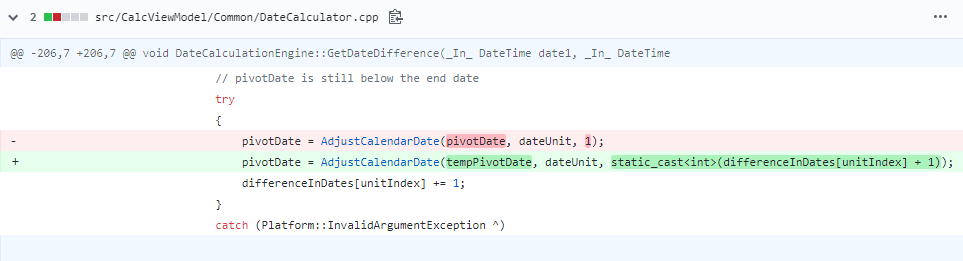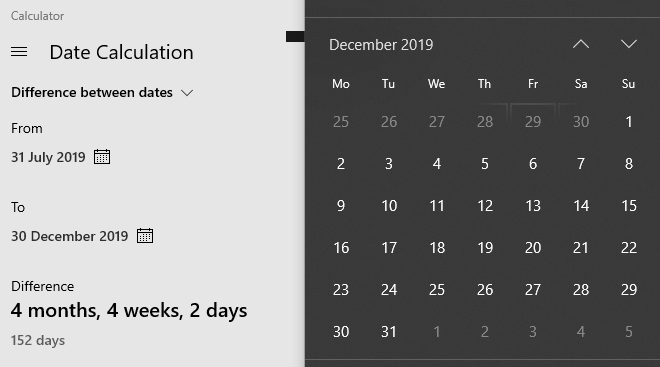﻿ 我为 Windows 10 修复了一个 bug - 代码学院 www.codexy.cn

# 我为 Windows 10 修复了一个 bug，Peter 决定从 下载源码来研究一番。从 repo 把源码下载到本地后，由于在 IDE 运行 Windows 计算器项目需要 UWP workload，所以 Peter 还为 添加了 UWP workload。不过 Peter 表示搭建开发环境也十分顺利，修 bug 第一步至此完成。

`DateDifference calculate_difference(start_date, end_date) { uint[] diff_types = [year, month, week, day] uint[] typical_days_in_type = [365, 31, 7, 1] uint[] calculated_difference = [0, 0, 0, 0] date temp_pivot_date date pivot_date = start_date uint days_diff = calculate_days_difference(start_date, end_date) for(type in differenceTypes) { temp_pivot_date = pivot_date uint current_guess = days_diff /typicalDaysInType[type] if(current_guess !=0) pivot_date = advance_date_by(pivot_date, type, current_guess) int diff_remaining bool best_guess_hit = false do{ diff_remaining = calculate_days_difference(pivot_date, end_date) if(diff_remaining < 0) { // pivotDate has gone over the end date; start from the beginning of this unit current_guess = current_guess - 1 pivot_date = temp_pivot_date pivot_date = advance_date_by(pivot_date, type, current_guess) best_guess_hit = true } else if(diff_remaining > 0) { // pivot_date is still below the end date if(best_guess_hit) break; current_guess = current_guess + 1 pivot_date = advance_date_by(pivot_date, type, 1) } } while(diff_remaining!=0) temp_pivot_date = advance_date_by(temp_pivot_date, type, current_guess) pivot_date = temp_pivot_date calculated_difference[type] = current_guess days_diff = calculate_days_difference(pivot_date, end_date) } calculcated_difference[day] = days_diff return calculcated_difference }`

Peter 表示这看起来很正常，他没发现其中的逻辑存在错误。

`date = advance_date_by(date, month, somenumber)date = advance_date_by(date, month, 1)`

`date = advance_date_by(date, month, somenumber + 1)`

“July 31st + 4 Months = November 30th”
“November 30th + 1 Month = December 30th”

“July 31st + 5 Months = December 31st”Peter 为修复加上了引号，是因为它最后计算出的结果如下：Peter 表示，如果各位认可“7月31日+ 4个月= 11月30日”这样的结果，他认为这在技术上是正确的。虽然完整的结果不符合大众对日期间隔天数的阅读习惯，但至少不会出错。